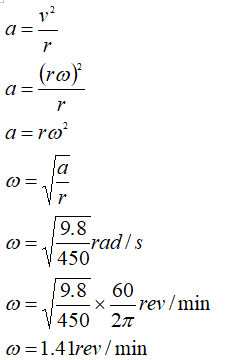# Diameter=900meters, a=9.8m/s^2, what is the revolution per minute?

Question
1 views

Diameter=900meters, a=9.8m/s^2, what is the revolution per minute?

check_circle

star
star
star
star
star
1 Rating
Step 1

Given

Acceleration a = 9.8 m/s2

Diameter D = 900 m

Radius r = D/2 = 450 m

Step 2

Solution...

### Want to see the full answer?

See Solution

#### Want to see this answer and more?

Solutions are written by subject experts who are available 24/7. Questions are typically answered within 1 hour.*

See Solution
*Response times may vary by subject and question.
Tagged in

### Physics